Frivolous Visualization Example: the Diving Board Beam

We wish to simulate the deflection of a diving board as a diver walks toward the end.  A diving board may be modeled as beam with constant cross-section. The boundary conditions are that the board is clamped at the beginning; has a pivot located somewhere near the center; and the "diving" end . For "competitive" diving boards, the pivot point is adjustable. Here we fix the pivot at x=1/2.

This next function is a silly little graphic for the walking diver.

Graphics Functions for Animating Board and Diver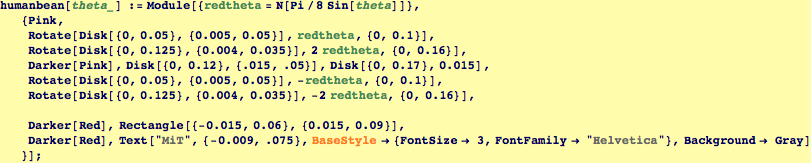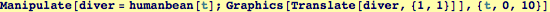The general solution for this beam equation is a bit complicated.  We approximate the diver's force with a Delta-function. To get a solution, we have to match boundary conditions at the center-pivot.  If the diver is to the left of the pivot, we use the implied slope at the pivot as input to the slope at the pivot for the beam to the right of the pivot.  If the diver is to the right of the pivot, we allow the slope to be an undetermined parameter, and then enforce that the shear force is continous across the pivot. The result is a fairly involved piece-wise function.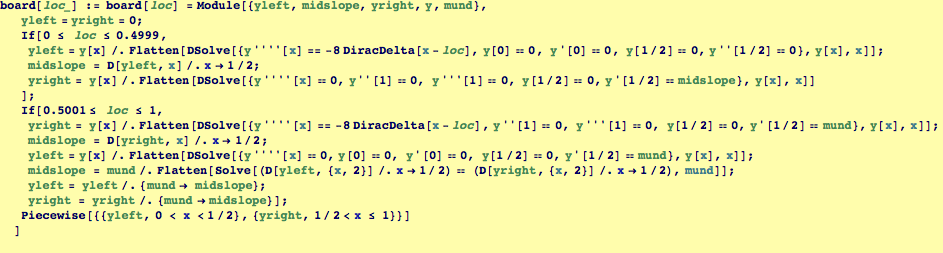This is a fixed graphic for the pivot.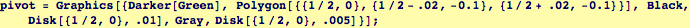And finally, our interactive simulation for a the walking diver.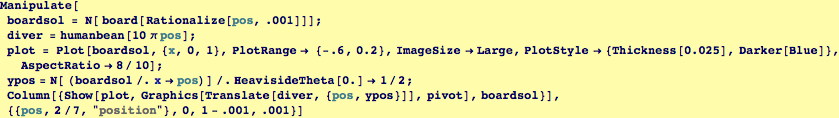Created by Wolfram Mathematica 6.0  (16 November 2007)